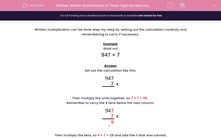# Written Multiplication of Three-Digit Numbers by One-Digit

In this worksheet, students must carry out formal written multiplications of three-digit numbers by single-digit numbers. Carrying is involved.This content is premium and exclusive to EdPlace subscribers.Key stage:  KS 2

Curriculum topic:   Maths and Numerical Reasoning

Curriculum subtopic:   Mixed Problems

Difficulty level:#### Worksheet Overview

Written multiplication can be done step-by-step by setting out the calculation carefully and remembering to carry if necessary.

Example

Work out

947 × 7

Set out the calculation like this:Then multiply the units together, so 7 × 7 = 49

Remember to carry the 4 tens below the next column.Then multiply the tens, so 4 × 7 = 28 and add the 4 that was carried.This gives us 28 + 4 = 32 , so we carry the 3 into the hundreds column and write the 2 in the tens column.Then multiply the hundreds, so 9 × 7 = 63 and add the 3 that was carried.

This gives us 63 + 3 = 66 in the thousands.So, in full, we have:So      947 × 7 = 6629

### What is EdPlace?

We're your National Curriculum aligned online education content provider helping each child succeed in English, maths and science from year 1 to GCSE. With an EdPlace account you’ll be able to track and measure progress, helping each child achieve their best. We build confidence and attainment by personalising each child’s learning at a level that suits them.

Get started